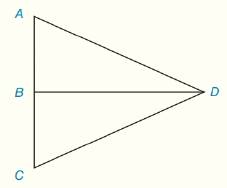Chapter 3.CR, Problem 21CR### Elementary Geometry for College St...

6th Edition
Daniel C. Alexander + 1 other
ISBN: 9781285195698

#### Solutions

Chapter
Section### Elementary Geometry for College St...

6th Edition
Daniel C. Alexander + 1 other
ISBN: 9781285195698
Textbook Problem
1 views

# G i v e n :         D B ¯ ⊥ A C ¯                                     A D ¯ ≅ D C ¯                                     m ∠ C = 70 ° F i n d     :           m ∠ A D BTo determine

Explanation

Procedure used:

If two sides of the triangle are equal then the corresponding angles are also equal in the triangle.

The sum of interior angles of the triangle is 180°.

Given:

The triangle ΔACD is shown in the figure below,

Figure (1)

From figure (1),

Calculation:

Consider the triangle ΔACD,

From the given data,

The segment DB¯ is perpendicular to the side AC¯, then the angle formed by the perpendicular is equal to right angle. So,

mABD=90°

Since, the side AD¯ is congruent to the side DC¯, then the congruent side have same measures. So,

Since, the two sides of the triangle are equal then the corresponding angles are also equal in the triangle

### Still sussing out bartleby?

Check out a sample textbook solution.

See a sample solution

#### The Solution to Your Study Problems

Bartleby provides explanations to thousands of textbook problems written by our experts, many with advanced degrees!

Get Started